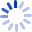# Perspectives in Computation

Computation is the process of applying a procedure or algorithm to the solution of a mathematical problem. Mathematicians and physicists have been occupied for many decades pondering which problems can be solved by which procedures, and, for those that can be solved, how this can most efficiently be done. In recent years, quantum mechanics has augmented our understanding of the process of computation and of its limitations. Perspectives in Computation covers three broad topics: the computation process and its limitations ...

##### Cash for Textbooks